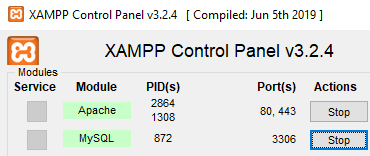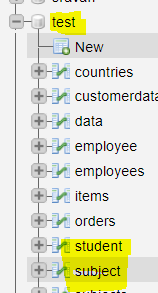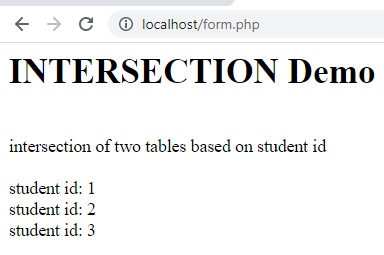Open In App

# PHP – MySQL : INTERSECTION operation

In this article, we are going to perform a database operation that includes an intersection of two tables using PHP in xampp server. So, we are taking the student database.

Requirements –xampp server

Introduction :

1. MySQL –
It is a database query language to manage databases. PHP is a server-side programming language to maintain and manipulate databases.

2. INTERSECTION –
It is a useful operator and is used to combine SELECT queries like if you have two SELECT statements then from the first SELECT it will return rows only and that will be identical to a row in the second SELECT statement.

Syntax :

```SELECT column1,column2,columnn
FROM table1
[WHERE condition]
INTERSECT
SELECT column1,column2,columnn
FROM table1 [, table2 ]
[WHERE condition];```

Example :
Consider the two tables as follows.

Table-1: Student table –

Table-2: Subject table –

Database Operation :
The intersection of two tables based on student id as follows.

```SELECT id FROM student
INTERSECT
SELECT sid FROM subject```

Result –

```student id: 1
student id: 2
student id: 3```

Approach :
Here, you will see the approach to implement the intersection operation as follows.

• Create database.
• Create tables.
• Write PHP code to insert records into the tables.
• Write PHP code to perform intersection operation.

Steps to implement intersection Operation :

• Start xampp server.• Create database named test in xampp server and tables named student and subject• Table structure will look as follows.

Table structure -student :

Table structure -subject :

PHP’s code to insert records into student table :
File name- student.php

## PHP

 `connect_error) {``  ``die``(``"Connection failed: "` `. ``\$conn``->connect_error);``}``//insert records into table``\$sql`  `= ``"INSERT INTO student VALUES (1,'sravan kumar',98,'kakumanu');"``;``\$sql` `.= ``"INSERT INTO student VALUES (2,'bobby',90,'kakumanu');"``;``\$sql` `.= ``"INSERT INTO student VALUES (3,'ojaswi',89,'hyderabad');"``;``\$sql` `.= ``"INSERT INTO student  VALUES (4,'rohith',90,'hyderabad');"``;``\$sql` `.= ``"INSERT INTO student  VALUES (5,'gnanesh',87,'hyderabad');"``;`` ` `if` `(``\$conn``->multi_query(``\$sql``) === TRUE) {``  ``echo` `"student data inserted successfully"``;``} ``else` `{``  ``echo` `"Error: "` `. ``\$sql` `. ``"
"` `. ``\$conn``->error;``}`` ` `\$conn``->close();``?>`

Output :Insert records into the subject table :
File name -subjects.php

## PHP

 `connect_error) {``  ``die``(``"Connection failed: "` `. ``\$conn``->connect_error);``}``//insert records into table``\$sql`  `= ``"INSERT INTO subject VALUES (1,'sravan kumar','Maths');"``;``\$sql` `.= ``"INSERT INTO subject  VALUES (7,'ramya','social');"``;``\$sql` `.= ``"INSERT INTO subject VALUES (2,'bobby','Maths');"``;``\$sql` `.= ``"INSERT INTO subject VALUES (3,'ojaswi','social');"``;``\$sql` `.= ``"INSERT INTO subject  VALUES (6,'ravi','hindi');"``;`` ` `if` `(``\$conn``->multi_query(``\$sql``) === TRUE) {``  ``echo` `"subject data inserted successfully"``;``} ``else` `{``  ``echo` `"Error: "` `. ``\$sql` `. ``"
"` `. ``\$conn``->error;``}`` ` `\$conn``->close();``?>`

Output :Now records in two tables are as follows.

Table-1: Subject table –

Table-2: Student table –

PHP code to perform intersection operation on id :
File name -form.php

## PHP

 `````"``; ``echo` `"INTERSECTION  Demo "``; ``echo``""``;``echo` `"
"``;``echo` `"intersection of two tables based on student id"``;``echo` `"
"``;``echo` `"
"``;``//sql query``\$sql` `= ``"SELECT id FROM student INTERSECT SELECT sid FROM subject "``;``\$result` `= ``\$conn``->query(``\$sql``);``//display data on web page``while``(``\$row` `= mysqli_fetch_array(``\$result``)){``    ``echo` `" student id: "``. ``\$row``[``'id'``];``    ``echo` `"
"``;``}`` ` `//close the connection`` ` `\$conn``->close();``?>`````

Output :
localhost/form.php